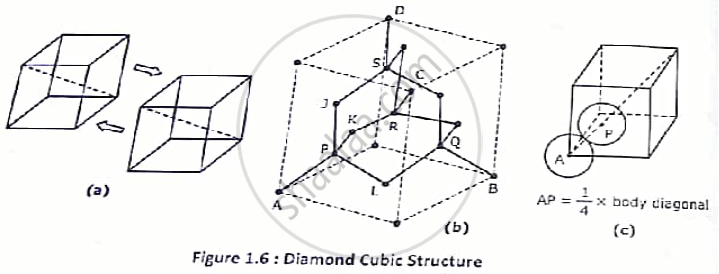BE Civil Engineering Semester 1 (FE First Year)University of Mumbai
Share
Notifications

View all notifications
Books Shortlist
Your shortlist is empty

# Find the following parameter for DC(Diamond Cubic) structure:- ⦁ No. of atoms per unit cell.⦁ Co-ordination number.⦁ Nearest atomic distance.⦁ Atomic radius.⦁ APF. - BE Civil Engineering Semester 1 (FE First Year) - Applied Physics 1

Login
Create free account

Forgot password?
ConceptStudy of characteristics of unit cell of Diamond, ZnS, NaCl and HCP;

#### Question

Find the following parameter for DC(Diamond Cubic) structure:-
⦁ No. of atoms per unit cell.
⦁ Co-ordination number.
⦁ Nearest atomic distance.
⦁ Atomic radius.
⦁ APF.

#### Solution

This can be thought of as a combination of two FCC sublattice as if one FCC sublattice is translated along a body diagonal of the other sublattice through a distance √3a/4 from one of its corners. Therefore in addition to regular FCC atoms additional atoms, one on each of the four body diagonals are found in this structure. Two FCC sublattice can form the DC structure.
⦁ NUMBER OF ATOMS/ UNIT CELL(n):-

Total number of atoms/ unit cell = (1/8 ×8 corners) +(1/2xx6face cornes ) +(4 × 1 on each body diagonal)

∴ n = 8⦁ CO-ORDINATION NUMBER(N)
Every atom with co-ordination (a/4, a/4, a/4) has four nearest neighbour, one at the nearest corner and three at the face centres of the three adjacent faces passing through the nearest corner. For example, the atom A, J, K and L are the nearest neighbours of the additional atom P. the five atoms A, J, K and P from a tetrahedron

∴ N = 4

⦁ ATOMIC RADIUS (r):-
The distance between two nearest neighbours is

AP =( √3a)/4 = 2r           ∴ r = (√3a)/8
⦁ ATOMIC PACKING FACTOR(APF):-

APF = (8xx(4π)/3(sqrt(3a)/8)^3)/(a^3)=0.34

Packing efficiency = 34%

Hence , it is a loosely packed structure. But as all the atoms are attached with covalent bonds this is a strong structure.
⦁ VOID SPACE:-
The void space in a DC unit cell is 66% of the entire cell structure.

Is there an error in this question or solution?

#### APPEARS IN

Solution Find the following parameter for DC(Diamond Cubic) structure:- ⦁ No. of atoms per unit cell.⦁ Co-ordination number.⦁ Nearest atomic distance.⦁ Atomic radius.⦁ APF. Concept: Study of characteristics of unit cell of Diamond, ZnS, NaCl and HCP;.
S